Processing ......FreeComputerBooks.com Links to Free Computer, Mathematics, Technical Books all over the World

Statistics, Mathematical Statistics, and SAS® Programming
Related Book Categories:
 Bayesian Thinking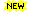Deep Learning and Neural Networks The R Programming LanguageData Analysis and Data Mining Numerical Analysis and Computing Calculus and Mathematical Analysis Probability and Stochastic Processes Machine LearningFinancial Mathematics and Engineering Applied Mathematics Algebra and Abstract Algebra Data Science•Foundations in Statistical Reasoning (Pete Kaslik)

This book is designed for students taking an introductory statistics class. The emphasis throughout the entire book is on how to make decisions with only partial evidence. It focuses on the thought process.

•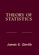Theory of Statistics (James E. Gentle)

This book is directed toward students for whom mathematical statistics is or will become an important part of their lives. It covers classical likelihood, Bayesian, and permutation inference; an introduction to basic asymptotic distribution theory; and modern topics.

•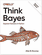O'Reilly® Think Bayes: Bayesian Statistics in Python

If you know how to program, you're ready to tackle Bayesian statistics. With this book, you'll learn how to solve statistical problems with Python code instead of mathematical formulas, using discrete probability distributions rather than continuous mathematics.

•Statistical Inference: Algorithms, Evidence, and Data Science

A masterful guide to how the inferential bases of classical statistics can provide a principled disciplinary frame for the data science of the twenty-first century. Every aspiring data scientist should carefully study this book, use it as a reference.

•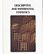Foundations of Descriptive and Inferential Statistics (H. van Elst)

This book aim to provide an accessible though technically solid introduction to the logic of systematical analyses of statistical data to both undergraduate and postgraduate students, in particular in the Social Sciences, Economics, and the Financial Services.

•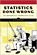Statistics Done Wrong: The Woefully Complete Guide (Reinhart)

Scientific progress depends on good research, and good research needs good statistics. But statistical analysis is tricky to get right, even for the best and brightest of us. You'd be surprised how many scientists are doing it wrong.

•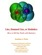Lies, Damned Lies: How to Tell the Truth with Statistics

The goal is to help you learn How to Tell the Truth with Statistics and, therefore, how to tell when others are telling the truth ... or are faking their "news". Covers Data Analysis, Binomial and normal models, Sample statistics, confidence intervals, hypothesis tests, etc.

•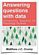Answering Questions with Data : Introductory Statistics

This is a free textbook teaching introductory statistics for undergraduates. Students will learn to select an appropriate data analysis technique, carry out the analysis, and draw appropriate conclusions.

•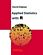Applied Statistics with R (David Dalpiaz)

This book provides an integrated treatment of statistical inference techniques in data science using the R Statistical Software. It provides a much-needed, easy-to-follow introduction to statistics and the R programming language.

•An Introduction to Statistical Learning: with Applications in R

It provides an accessible overview of the field of statistical learning, an essential toolset for making sense of the vast and complex data sets that have emerged in fields ranging from biology to finance to marketing to astrophysics in the past twenty years.

•The Elements of Statistical Learning: Data Mining, Inference, etc.

This book describes the important ideas in these areas in a common conceptual framework. While the approach is statistical, the emphasis is on concepts rather than mathematics. Many examples are given, with a liberal use of color graphics.

•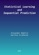Statistical Learning and Sequential Prediction

This book focuses on theoretical aspects of Statistical Learning and Sequential Prediction, a unified approach to analyzing learning in both scenarios, brings together ideas from probability and statistics, game theory, algorithms, and optimization.

•Statistical Methodologies and Their Application to Real Problems

This book provides a cross-disciplinary forum for exploring the variety of new data analysis techniques emerging from different fields, focusing on recent advances in data analysis techniques in many different research fields.

•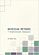Bayesian Methods for Statistical Analysis (Borek Puza)

Bayesian methods for statistical analysis is a book on statistical methods for analysing a wide variety of data. It contains many exercises, all with worked solutions, including complete computer code.

•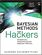Bayesian Methods for Hackers: Probabilistic Programming

This book illuminates Bayesian inference through probabilistic programming with the powerful PyMC language and the closely related Python tools NumPy, SciPy, Matplotlib, through practical examples and computation - no advanced mathematics required.

•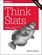Think Stats, 2nd Edition: Exploratory Data Analysis in Python

This concise introduction shows you how to perform statistical analysis computationally, rather than mathematically, with programs written in Python. You'll become familiar with distributions, the rules of probability, visualization, and many other tools and concepts.

•Introduction to the Theory of Statistics (Alexander M. Mood, et al)

This book is a self contained introduction to classical statistical theory. The material is suitable for students who have successfully completed a single year's course in calculus with no prior knowledge of statistics or probability.

•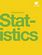Introductory Statistics (OpenStax College)

This book is geared toward students majoring in fields other than math or engineering. This text assumes students have been exposed to intermediate algebra, and it focuses on the applications of statistical knowledge rather than the theory behind it.

•Seeing Theory: A Visual Introduction to Probability and Statistics

The goal of this book is to make statistics more accessible through interactive visualizations (using D3.js). It visualizes the fundamental concepts covered in an introductory college statistics or Advanced Placement statistics class.

•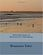Introduction to Statistical Thinking, with R, without Calculus

This is an introduction to statistics, with R, without calculus, for students who are required to learn statistics, students with little background in mathematics and often no motivation to learn more.

•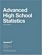Advanced High School Statistics (David M Diez, et al)

This book is geared to the high school audience and is specifically tailored to be aligned with the AP Statistics curriculum. It is already being used by many high schools and community colleges throughout the country.

•Statistics Fundamentals Succinctly (Katie Kormanik)

This book provides the foundational bricks and mortar needed to master the theories and methodologies behind statistical procedures. In just a little more than 100 pages, you'll understand how to better gather and interpret all the information at your fingertips.

•Collaborative Statistics (Barbara Illowsky, et al)

This book is intended for introductory statistics courses being taken by students at two- and four-year colleges who are majoring in fields other than math or engineering. Intermediate algebra is the only prerequisite.

•Introduction to Statistical Thought (Michael Lavine)

The book is intended as an upper level undergraduate or introductory graduate textbook in statistical thinking with a likelihood emphasis for students with a good knowledge of calculus and the ability to think abstractly.

•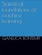Statistical Foundations of Machine Learning: The Handbook

This book aims to present the statistical foundations of machine learning intended as the discipline which deals with the automatic design of models from data. All the examples are implemented in the statistical programming language R.

•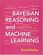Bayesian Reasoning and Machine Learning (David Barber)

This practical introduction is ideally suited to computer scientists without a background in calculus and linear algebra. You'll develop analytical and problem-solving skills that equip them for the real world. Numerous examples and exercises are provided.

•A Survey of Statistical Network Models (Anna Goldenberg, et al.)

This book aims to provide the reader with an entry point to the voluminous literature on statistical network modeling. It guides the reader through the development of key stochastic network models, touches upon a number of examples and commonalities.

•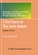A First Course on Time Series Analysis with SAS (Michael Falk, et al)

A unique feature of this book is its integration with the statistical software package SAS® (Statistical Analysis System) computing environment. Basic applied statistics is assumed through multiple regression.

•Step-By-Step Programming With Base SAS® Software

This book provides conceptual information about SAS® software along with step-by-step examples that illustrate the concepts. It answers every question that a new or intermediate SAS® user might have. Examples are thorough, readable, and clear.

•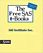Free SAS® e-Books (SAS Institute, Inc.)

Gain insight on SAS® solutions and analytics technology with a collection of free e-books, includes Artificial Intelligence, Machine Learning, Computer Vision, Natural Language Processing, Programming for R Users, etc.

•Applied Statistics (Mohammed A. Shayib)

This book is intended to introduce the concepts, definitions, and terminology of the subject in an elementary presentation with minimum mathematical background which does not surpass college algebra.

•Statistical Inference for Everyone (Brian S Blais)

Approaching an introductory statistical inference textbook in a novel way, this book walks through a simple introduction to probability, and then applies those principles to all problems of inference.

•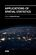Applications of Spatial Statistics (Ming-Chih Hung)

Spatial statistics has been widely used in many environmental studies. This book is for people who are interested in applying statistics to spatial data, as well as for who know statistics and want to explore how it can be applied to spatial data.

•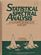Statistical Spectral Analysis: A Non-Probabilistic Theory

This book is intended to serve as both a graduate-level textbook and a technical reference. The focus is on fundamental concepts, analytical techniques, and basic empirical methods. The only prerequisite is an introductory course on Fourier analysis.

•Introduction to Probability, Statistics, and Random Processes

This book introduces students to probability, statistics, and stochastic processes. It provides a clear and intuitive approach to these topics while maintaining mathematical accuracy.

•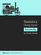Statistics Using Excel Succinctly (Charles Zaiontz)

This book illustrates the capabilities of Microsoft Excel to teach applied statistics effectively. It is a step-by-step exercise-driven guide for students and practitioners who need to master Excel to solve practical statistical problems

•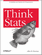O'Reilly® Think Stats: Probability and Statistics for Programmers

With this book, you'll learn how to solve statistical problems with Python code instead of mathematical notation, and use discrete probability distributions instead of continuous mathematics.

•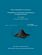From Algorithms to Z-Scores: Probabilistic and Statistical Modeling

This is a textbook for a course in mathematical probability and statistics for computer science students. Computer science examples are used throughout, in areas such as: computer networks; data and text mining; computer security; etc.

•StatLect - Lectures on Probability Theory and Statistics

This is a collection of lectures on probability theory and mathematical statistics. It is offered as a free service to the mathematical community and provides an accessible introduction to topics that are not usually found in elementary textbooks.

•Probability Theory and Mathematical Statistics (Prasanna Sahoo)

This book is both a tutorial and a textbook. It presents an introduction to probability and mathematical statistics and it is intended for students already having some elementary mathematical background.

•Probability and Statistics: A Course for Physicists and Engineers

This book offers an introduction to concepts of probability theory, probability distributions relevant in the applied sciences, and focuses on real engineering applications, as well as basics of sampling distributions, estimation and hypothesis testing.

•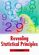Revealing Statistical Principles (Jim K. Lindsey)

Written by a renowned statistician, this book presents the basic ideas behind the statistical methods commonly used in studies of human subjects. Accessible to a wide audience with little background in statistics.

•Introduction to Probability and Statistics Using R (G. Jay Kerns)

This is a textbook for an undergraduate course in probability and statistics. Calculus and some linear algebra knowledge is required.

•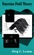Bayesian Field Theory (Jorg C. Lemm)

Long the province of mathematicians and statisticians, Bayesian methods are applied in this ground-breaking book to problems in cutting-edge physics, with practical examples of Bayesian analysis for the physicist working in such areas as neural networks, artificial intelligence, and inverse problems in quantum theory.

•Learning Statistics with R (Daniel Navarro)

This book takes you on a guided tour of software development with R, from basic types and data structures to advanced topics like closures, recursion, and anonymous functions. No statistical knowledge is required.

•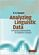Analyzing Linguistic Data: Introduction to Statistics using R

A straightforward introduction to the statistical analysis of language, designed for those with a non-mathematical background. Using the leading statistics programme 'R'. Suitable for all those working with quantitative language data.

•Statistics with R (Vincent Zoonekynd)

It provides an elementary-level introduction to R, targeting both non-statistician scientists in various fields and students of statistics, via code examples with liberal commenting of the code and the output, from the computational and the statistical viewpoint.

•Gaussian Processes for Machine Learning (Carl E. Rasmussen)

This book provides a long-needed systematic and unified treatment of theoretical and practical aspects of Gaussian Processes (GPs) in machine learning. It deals with the supervised-learning problem for both regression and classification.

•Nonparametric and Semiparametric Models (Wolfgang K. Hardle)

The aim of this book is to present the statistical and mathematical principles of smoothing with a focus on applicable techniques. The necessary mathematical treatment is easily understandable and a wide variety of interactive smoothing examples are given.

•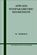Applied Nonparametric Regression (Wolfgang K. Hardle)

This book focuses on the applications and practical problems of two central aspects of curve smoothing: the choice of smoothing parameters and the construction of confidence bounds.

•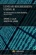Linear Regression Using R: An Introduction to Data Modeling

This book presents one of the fundamental data modeling techniques in an informal tutorial style. Learn how to predict system outputs from measured data using a detailed step-by-step process to develop, train, and test reliable regression models.

•Applied Multivariate Statistical Analysis (Wolfgang K. Hardle)

This book gives a broad and modern introduction to statistics for multivariate data. A bunch of interesting examples is used to illustrate the techniques.

•Theory and Applications of Monte Carlo Simulations (Wai Kin Chan)

The purpose of this book is to introduce researchers and practitioners to recent advances and applications of Monte Carlo Simulation (MCS). Random sampling is the key of the MCS technique.

•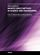Applications of Monte Carlo Method in Science and Engineering

This book exposes the broad range of applications of Monte Carlo simulation in the fields of Quantum Physics, Statistical Physics, Reliability, Medical Physics, Polycrystalline Materials, Ising Model, Chemistry, Agriculture, Food Processing, X-ray Imaging, ...

•Statistical Tools for Finance and Insurance (Pavel Cizek, et al)

This book presents ready-to-use solutions, theoretical developments and method construction for many practical problems in quantitative finance and insurance.

•A Handbook of Statistical Analyses Using R (Brian S. Everitt, et al)

This book is the perfect guide for newcomers as well as seasoned users of R who want concrete, step-by-step guidance on how to use the software easily and effectively for nearly any statistical analysis.

•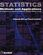Statistics: Methods and Applications (Thomas Hill, et al)

This book offers a comprehensive, almost encyclopedic presentation of statistical methods and analytic approaches used in science, industry, business, and data mining, written from the perspective of the real-life practitioner ('consumer') of these methods.

•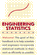Engineering Statistics Handbook (NIST/SEMATECH)

The goal of this handbook is to help scientists and engineers incorporate statistical methods in their work as efficiently as possible.

•Introductory Statistics (T. H. Wonnacott, et al)

This book is an introduction to statistics for students of economics or business, suitable for a one or two-semester course, yet uses the simplest mathematics consistent with a sound presentation.

•Natural Image Statistics in Digital Image Forensics (Siwei Lyu)

This book provides the first general framework, based on universal statistical properties of natural images, of detecting tampering and authenticating digital images that has been successfully applied to three problems in digital image forensics.

•Introduction to Statistical Signal Processing (Robert M. Gray, et al)

This book describes the essential tools and techniques of statistical signal processing, with specific applications to the analysis of random signals and systems for communicating, estimating, detecting, modulating, etc.

•Window Functions and Their Applications in Signal Processing

This book presents an exhaustive and detailed account of Window Functions and their applications in signal processing, focusing on the areas of digital spectral analysis, design of FIR filters, pulse compression radar, and speech signal processing.

•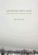Modeling with Data: Tools and Techniques for Scientific Computing

Modeling with Data fully explains how to execute computationally intensive analyses on very large data sets, showing readers how to determine the best methods for solving a variety of different problems, etc..

•Machine Learning, Neural and Statistical Classification (D. Michie)

Statistical, machine learning and neural network approaches to classification are all covered in this book to provide an objective assessment of the potential for machine learning algorithms in solving significant commercial and industrial problems, widening the foundation for exploitation of these and related algorithms.

•A Practical Guide to Geostatistical Mapping (Tomislav Hengl)

A practical guide to geostatistical mapping using R+gstat/geoR, SAGA GIS and Google Earth combo of software packages.

•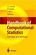Handbook of Computational Statistics: Concepts and Methods

This handbook cover the important subareas of computational statistics and give some flavor of the wide range of applications.

•Using R for Introductory Statistics (John Verzani)

This book lays the foundation for further study and development in statistics using R - an ideal text for integrating the study of statistics with a powerful computational tool.

•Python for Econometrics, Statistics and Data Analysis

This book provides an introduction to Python for a beginning programmer. They may also be useful for an experienced Python programmer interested in using NumPy, SciPy, and matplotlib for numerical and statistical analaysis.

•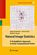Natural Image Statistics: A Probabilistic Approach (A. Hyvarinen)

This book is both an introductory textbook and a research monograph on modelling the statistical structure of natural images. The statistical structure is described using a number of statistical models whose parameters are estimated from image samples.

Book Categories
 :All CategoriesRecent BooksMiscellaneous BooksComputer LanguagesComputer ScienceData Science/DatabasesElectrical EngineeringJava and Java EE (J2EE)Linux and UnixMathematicsMicrosoft and .NETMobile ComputingNetworking and CommunicationsSoftware EngineeringSpecial TopicsWeb Programming
Other Categories